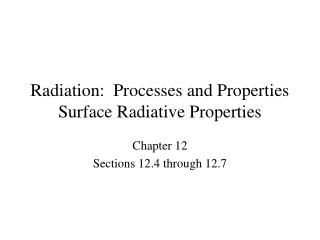DownloadDownload PresentationTélécharger la présentation- - - - - - - - - - - - - - - - - - - - - - - - - - - E N D - - - - - - - - - - - - - - - - - - - - - - - - - - -
##### Presentation Transcript

1. Radiation: Processes and PropertiesSurface Radiative Properties Chapter 12 Sections 12.4 through 12.7

2. Surface Emissivity Emissivity • Radiation emitted by a surface may be determined by introducing a property • (the emissivity) that contrasts its emission with the ideal behavior of a blackbody • at the same temperature. • The definition of the emissivity depends upon one’s interest in resolving • directional and/or spectral features of the emitted radiation, in contrast • to averages over all directions (hemispherical and/or wavelengths (total). • The spectral, directional emissivity: • The spectral, hemispherical emissivity (a directional average):

3. Emissivity (cont) • The total, hemispherical emissivity (a directional and spectral average): • To a reasonable approximation, the hemispherical emissivity is equal to • the normal emissivity. • Representative values of the total, normal emissivity: • Note: • Low emissivity of polished metals and increasing emissivity for unpolished and oxidized surfaces. • Comparatively large emissivities of nonconductors.

4. Note decreasing with increasing for metals and different behavior for nonmetals. Why does increase with increasing for tungsten and not for aluminum oxide? Emissivity (cont) • Representative spectral variations: • Representative temperature variations:

5. Reflection from the medium • Absorption within the medium • Transmission through the medium • In contrast to the foregoing volumetric effects, the response of an opaque material • to irradiation is governed by surface phenomena and Radiation balance Abs, Ref & Trans Response to Surface Irradiation: Absorption, Reflection and Transmission • There may be three responses of a semitransparentmedium to irradiation: • The wavelength of the incident radiation, as well as the nature of the material, • determine whether the material is semitransparent or opaque. • Are glass and water semitransparent or opaque?

6. Abs, Ref & Trans (cont) • Unless an opaque material is at a sufficiently high temperature to emit visible • radiation, its color is determined by the spectral dependence of reflection in • response to visible irradiation. • What may be said about reflection for a white surface? A black surface? • Why are leaves green?

7. The absorptivity is approximately independent of the surface temperature, but if the irradiation corresponds to emission from a blackbody, why does depend on the temperature of the blackbody? Absorptivity of an Opaque Material Absorptivity • The spectral, directional absorptivity: Assuming negligible temperature dependence, • The spectral, hemispherical absorptivity: • To what does the foregoing result simplify, if the irradiation is diffuse? If the surface is diffuse? • The total, hemispherical absorptivity: • If the irradiation corresponds to emission from a blackbody, how may the above expression be rewritten?

8. Reflectivity of an Opaque Material Reflectivity • The spectral, directional reflectivity: Assuming negligible temperature • dependence: • The spectral, hemispherical reflectivity: • To what does the foregoing result simplify if the irradiation is diffuse? If the surface is diffuse? • The total, hemispherical reflectivity: • Limiting conditions of diffuse and • spectral reflection. Polished and rough surfaces.

9. Note strong dependence of Reflectivity (cont) • Is snow a highly reflective substance? White paint?

10. Transmissivity • The spectral, hemispherical transmissivity: Assuming negligible temperature dependence, Transmissivity Note shift from semitransparent to opaque conditions at large and small wavelengths. • The total, hemispherical transmissivity: • For a semitransparent medium,

11. Kirchhoff’s Law Kirchhoff’s Law • Kirchhoff’s law equates the total, hemispherical emissivity of a surface to its • total, hemispherical absorptivity: However, conditions associated with its derivation are highly restrictive: Irradiation of the surface corresponds to emission from a blackbody at the same temperature as the surface. • However, Kirchhoff’s law may be applied to the spectral, directional properties • without restriction: Why are there no restrictions on use of the foregoing equation?

12. With and Under what conditions may we equate • With and Under what conditions may we equate Diffuse/Gray Surfaces Diffuse/Gray Surfaces • Conditions associated with • assuming a gray surface:

13. Problem 12.49: Determination of the solar absorptivity and total emissivity of a diffuse surface from knowledge of the spectral distribution of and the surface temperature. Problem: Surface Emissivity and Absorptivity

14. Problem: Surface Emissivity and Absorptivity (cont)

15. Problem: Surface Emissivity and Absorptivity (cont)

16. Problem: Energy Balance for an Irradiated Surface Problem 12.90: Determination of the emissivity and absorptivity of a coated vertical plate exposed to solar-simulation lamps and the magnitude of the irradiation required to maintain a prescribed plate temperature.

17. Problem: Energy Balance for an Irradiated Surface (cont)

18. Problem: Energy Balance for an Irradiated Surface (cont)

19. Problem: Energy Balance for an Irradiated Surface (cont)